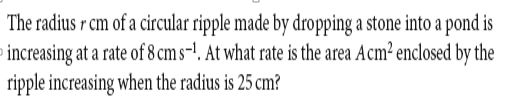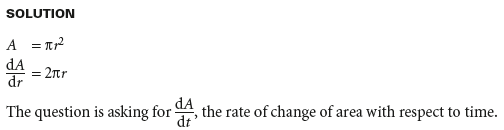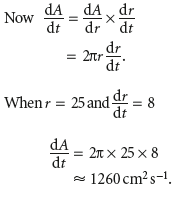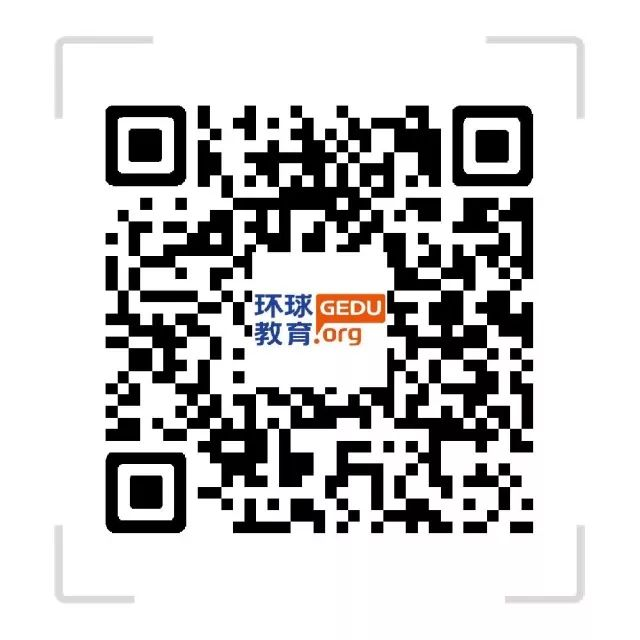# Alevel数学干货，微积分之链式法则

Alevel数学干货，微积分之链式法则1.The China rule (链式法则)

If y=f(u) is a differentiable function of u and u=g(x) is a differentiable function of x, then y=f(g(x)) is a differentiable function of x and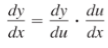or, equivalently,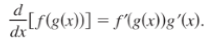Example 1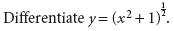SOLUTION

As you saw earlier, you can break down this expression as follows.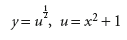Differentiation these gives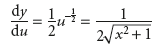and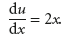By the chain rule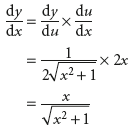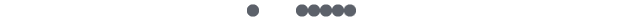2. Related-Rate Problems

Differentiation with respect to different variables

The chain rule makes it possible to differentiate with respect to a variable which does not feature in the original expression. For example, the volume V of a sphere of radius r is given by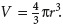Differentiating this with respect to r gives the rate of change of volume with radius,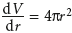However you might be more interested in finding dv/dt, the rate of change of change of volume with time, t. To find this, you would use the chain rule: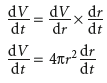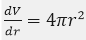Example 2